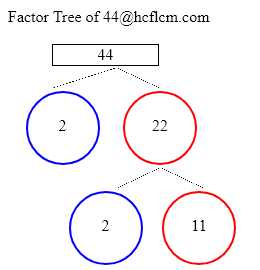# Factors of 44 | Find the Factors of 44 by Factoring Calculator

Factoring Calculator calculates the factors and factor pairs of positive integers.

Factors of 44 can be calculated quickly with the help of Factoring Calculator i.e. 1, 2, 4, 11, 22, 44 positive integers that divide 44 without a remainder.

Factors of 44 are 1, 2, 4, 11, 22, 44. There are 6 integers that are factors of 44. The biggest factor of 44 is 44.

Factors of:

### Factor Tree of 44 to Calculate the Factors

 44 2 22 2 11Factors of 44 are 1, 2, 4, 11, 22, 44. There are 6 integers that are factors of 44. The biggest factor of 44 is 44.

Positive integers that divides 44 without a remainder are listed below.

• 1
• 2
• 4
• 11
• 22
• 44

• 1 × 44 = 44
• 2 × 22 = 44
• 4 × 11 = 44
• 11 × 4 = 44
• 22 × 2 = 44
• 44 × 1 = 44

### Factors of 44 Table

FactorFactor Number
1one
2two
4four
11eleven
22twenty two
44forty four

### How to find Factors of 44?

As we know factors of 44 are all the numbers that can exactly divide the number 44 simply divide 44 by all the numbers up to 44 to see the ones that result in zero remainders. Numbers that divide without remainder are factors and in this case below are the factors

1, 2, 4, 11, 22, 44 are the factors and all of them can exactly divide number 44.

### Frequently Asked Questions on Factors of 44

1. What are the factors of 44?

Answer: Factors of 44 are the numbers that leave a remainder zero. The ones that can divide 44 exactly i.e. factors are 1, 2, 4, 11, 22, 44.

2.What are Factor Pairs of 44?